Full Text:<4478>

CLC number: P743

On-line Access: 2013-08-01

Revision Accepted: 2013-05-20

Crosschecked: 2013-07-10

Cited: 4

Clicked: 8423

Citations:  Bibtex RefMan EndNote GB/T7714

 Journal of Zhejiang University SCIENCE A 2013 Vol.14 No.8 P.574-582 http://doi.org/10.1631/jzus.A1300082

Three-dimensional numerical simulation of a vertical axis tidal turbine using the two-way fluid structure interaction approach*

 Author(s):  Syed-shah Khalid, Liang Zhang, Xue-wei Zhang, Ke Sun Affiliation(s):  . Deepwater Engineering Research Center, Harbin Engineering University, Heilongjiang 150001, China Corresponding email(s):   shahkhalidshah@yahoo.com Key Words:  Vertical axis tidal turbine, Renewable energy, Two-way fluid structure interaction (FSI) Share this article to： More <<< Previous Article|Next Article >>>

Syed-shah Khalid, Liang Zhang, Xue-wei Zhang, Ke Sun. Three-dimensional numerical simulation of a vertical axis tidal turbine using the two-way fluid structure interaction approach[J]. Journal of Zhejiang University Science A, 2013, 14(8): 574-582.

@article{title="Three-dimensional numerical simulation of a vertical axis tidal turbine using the two-way fluid structure interaction approach",
author="Syed-shah Khalid, Liang Zhang, Xue-wei Zhang, Ke Sun",
journal="Journal of Zhejiang University Science A",
volume="14",
number="8",
pages="574-582",
year="2013",
publisher="Zhejiang University Press & Springer",
doi="10.1631/jzus.A1300082"
}

%0 Journal Article
%T Three-dimensional numerical simulation of a vertical axis tidal turbine using the two-way fluid structure interaction approach
%A Syed-shah Khalid
%A Liang Zhang
%A Xue-wei Zhang
%A Ke Sun
%J Journal of Zhejiang University SCIENCE A
%V 14
%N 8
%P 574-582
%@ 1673-565X
%D 2013
%I Zhejiang University Press & Springer
%DOI 10.1631/jzus.A1300082

TY - JOUR
T1 - Three-dimensional numerical simulation of a vertical axis tidal turbine using the two-way fluid structure interaction approach
A1 - Syed-shah Khalid
A1 - Liang Zhang
A1 - Xue-wei Zhang
A1 - Ke Sun
J0 - Journal of Zhejiang University Science A
VL - 14
IS - 8
SP - 574
EP - 582
%@ 1673-565X
Y1 - 2013
PB - Zhejiang University Press & Springer
ER -
DOI - 10.1631/jzus.A1300082

Abstract:
The objective of this study was to develop, as well as validate the strongly coupled method (two-way fluid structural interaction (FSI)) used to simulate the transient FSI response of the vertical axis tidal turbine (VATT) rotor, subjected to spatially varying inflow. Moreover, this study examined strategies on improving techniques used for mesh deformation that account for large displacement or deformation calculations. The blade’s deformation for each new time step is considered in transient two-way FSI analysis, to make the design more reliable. Usually this is not considered in routine one-way FSI simulations. A rotor with four blades and 4-m diameter was modeled and numerically analyzed. We observed that two-way FSI, utilizing the strongly coupled method, was impossible for a complex model; and thereby using ANSYS-CFX and ANSYS-MECHANICAL in work bench, as given in ANSYS-WORKBENCH, helped case examples 22 and 23, by giving an error when the solution was run. To make the method possible and reduce the computational power, a novel technique was used to transfer the file in ANSYS-APDL to obtain the solution and results. Consequently, the results indicating a two-way transient FSI analysis is a time- and resource-consuming job, but with our proposed technique we can reduce the computational time. The ANSYS STRUCTURAL results also uncover that stresses and deformations have higher values for two-way FSI as compared to one-way FSI. Similarly, fluid flow CFX results for two-way FSI are closer to experimental results as compared to one-way simulation results. Additionally, this study shows that, using the proposed method we can perform coupled simulation with simple multi-node PCs (core i5).

## 1.  Introduction

The growing costs and highly fluctuating prices of oil and natural gas, as well as their constantly diminishing supplies globally, have created the need for less expensive and sustainable alternative energy resources.

Tidal turbines that harvest tide energy and convert it to electrical power are an alternative energy source, playing an increasingly prominent role globally, while receiving much attention from governments, industry, and researchers. There are two major classifications of tidal turbines: horizontal axis (HA) and vertical axis tidal turbine (VATT). This classification is based on the turbine axis with respect to water flow. VATT has several advantages, but the design and the prediction of its hydrodynamic behavior is more intricate (Paraschivoiu, ; Zanette et al., ). A key issue during the design phase is the evaluation of a turbine both in numerical simulation and experimental tests. Due to the high cost of experimental tests, emphases have been placed on developing sophisticated simulation methods, particularly since the 1980s, when high-speed computational technologies became widely accessible. Numerical models may be 1D, 2D, or 3D. Of course, 3D models have the capability of being more accurate and reliable as compared to 2D models, but the computational cost will be higher (Li and Calisal, ).

The VATT was subjected to a severe fatigue loading regime. The blades were submitted for a complete reversal stress cycle at each revolution. The entire pressure loading was placed on the blades. The blades transferred these forces to the shaft via connecting arms. Therefore, the performance of the turbine, while at the same time minimizing the cost of manufacturing the design, usually strived for thinner blades. Since high-stress levels produce deflection in the blade which obviously changes the velocity profile, we decided to ignore these deformations in simple one-way FSI simulations. Thus, establishing a more accurate finite element analysis (FEA) simulation is vital.

Previously, theoretical and numerical calculations did not take into account the deformation in structure due to fluid pressure, and an independent simulation was completed for fluid and structure/solid domains (Zhang et al., ; Calcagno et al., ). Furthermore, Dobrev and Massouh () applied this hybrid scheme for a wind turbine rotor using both 3D and 2D models. They showed that using a mixed model can save computational time. Moreover, a loosely coupled FSI scheme was used by Dang et al. () for an aeroelastic wing problem for high aspect ratio using constant volume tetrahedron interfacing technique for coupling computational fluid dynamics (CFD) and computational structural dynamics (CSD). For a wind turbine, FSI scheme was used by Kim and Kim (). In the field of aerospace, FSI theory was used by Garelli et al. () for rocket engine nozzle and for aerofoil by Ramji and Wei (). Recently, Jo et al. () conducted the loosely coupled method to compute the deformation in pile structure of a wind turbine. For the 2D model, a similar study was performed for wind turbine blades by Ouahiba et al. (). A VATT can be used anywhere where the water has sufficient current velocity to rotate the rotor, such as in an estuary, sea, river, or channel. From the previous studies mentioned above, we can find the importance of the FSI technique, and to the best of our knowledge, this technique is not used yet for VATT.

The main purpose of this study was to use a strongly coupled (two-way FSI) method to analyze the rotor of VATT for the maximum coefficient of performance C P. In this study, the CSD obtains hydrodynamic loadings through fluid solid interface at the blade surfaces from ANSYS mechanical applica-tion (MA). Centrifugal force and gravity are defined by rotational velocity and gravitation acceleration. The deformed shape form CSD is further coupled with CFD, from which new loadings are determined. This iterative process is continued until a suitable level of convergence is achieved. Obviously, the two-way FSI solution takes more computational power and time due to the strong coupling scheme. However, using a new technique of file sharing, we can reduce this simulation time. In this method, a file (ds.dat file) was generated in ANSYS-MECHANICAL after defining physics and boundary conditions in the model, and saved in a separate folder. Later, during CFX-Pre analysis, we imported ds.dat file and ran this in CFX solver. This requires an equal number of timestep and size in MA and CFX. An outer connection was made between the CFX and structure solver. Finally, we obtained the structural results (stress and deformations) in ANSYS-APDL. Using this file sharing and remote connection technique, we can reduce the simulation run time for the same number of iterations. Furthermore, the coupling between CFD/CSD was impossible for complex geometry without generation of this file in ANSYS-WORKBENCH. Thus, file shairing technique can be applied with a simple icore computer when using ANSYS-WORKBENCH platform for an FSI problem.

## 2.  Mathematical modeling and background

The Navier-Stokes equation becomes unstable when the Reynolds number is greater than one (Re>1). Engery is transferred from large eddies to the smaller ones, called Kolmogorov scale where eddies are dissipated by viscous forces: , where l K is the Kolmogorov scale, L is the length of the wall. Thus, the direct numerical simulation (DNS) computes all relevant scales up to the Kolmogorov scale. Often DNS is impossible, so Reynolds average Navier-Stokes (RANS) in combination with turbulence models can be expressed as (Menter, ; Wei et al., ; Fabio, ) , , where xi (i=1, 2, 3) is the Cartesian coordinate, ν is the kinematic viscosity of water, ui is the velocity component in the corresponding direction, p is the pressure, ρ is the density of the fluid, and fi represents an external body force field. Moreover, the modeling was based on the shear stress transport model (SST) (Menter, ), which is composed of the k-ω and k-ε models. Consequently, turbulent kinetic energy (k) and turbulent kinetic energy dissipation rate (ε) equations can be written as , , where ω is the turbulent dissipation rate, ∇ can be written as , α=0.44, β=0.0828, and μ is the molecular dynamic viscosity. U is the velocity vector as . Pk t3S U , , μ t is the turbulent eddy viscosity, , where , and Ω is the vorticity magnitude.

The SST model is defined as , where ϕ 1 represents the basic k-ω model, and ϕ 2 represents the modified k-ω model. is a blending function, and , where y is the distance from the field point to the nearest wall. F 1=1 in the near-wall region, and F 1=0 in the outer region , , , and is the turbulent kinematic viscosity. After solving all these equations, the Navier equation can be rewritten as , and we can further simplify Eq. (7) by ignoring the body forces as , where λ and μ are Lame constants as mentioned above. The values of various constants are: β=0.09, α=0.44, σk=1, and σω=1.168. The above numerical equations were solved based on the basic CFD codes using pressure-velocity coupling. A pressure was computed at the cell centers, and velocities were computed at cell faces. Moreover, the finite volume method (FVM) was used to discretize the fluid equations, and the second-order upwind discretization scheme was employed for convection term; the Newmark method was used for vibration equation. FVM was used for heterogeneous material, and also could handle discontinuities in solution. Another advantage of FVM over the finite difference method (FDM) was that it did not require structure mesh (Hyman et al., ). Casadei et al. () conducted the FSI technique for different 2D and 3D models using the mathematical algorithm for non-liner cases.

## 3.  Fluid structure interaction (FSI)

FSI is a two field problem: one fluid flow and one structural field. The fields are interfaced via the so-called wet surface where the pressure and friction forces produced by the fluid are acting on the structure. Due to these pressure loads, the body becomes deformed, and changes the boundaries of the fluid domain, as well as the flow pattern. Numerically, these two fields are coupled via a third field, the numerical meshes. The structural deformations are transferred through the fluid via adapting the mesh representing the fluid domain (Hubner et al., ).

FSI solution strategies can be divided into monolithic and partitioned methods. In this scheme, we try to solve the non-liner problem all at once. In the partitioned strategy, we also iteratively solve the fluid and structure subproblems. This requires two codes: one for solving the fluid equations and the other for structure problems. The partitioned method is further divided into two major categories: one-way and two-way coupling. Two-way can be further divided into a loose (weak) and strongly coupled method. Weak and strong couplings are distinguished by finite element formulation techniques used to develop the matrix equations. This method responds after every one-iteration for a coupled solution. Minimally, two iterations are required to achieve a coupled response for weak coupling (Vaassen et al., ; Benra et al., ). FSI problems can be solved using one-way or two-way FSI techniques.

In one-way FSI, the fluid field is solved only for the pressure forces, and these pressure forces are later applied on the body in solid or structure analyses in ANSYS to obtain the stresses. The flow field altered due to structural deformation when CFX analysis is ignored. It is also assumed that no displacement/deformation is produced during CFX analysis. While in two-way FSI, the pressure forces as well as displacement produced when CFX analysis is transferred to the structural analysis in ANSYS for stresses, and ANSYS results for pressure forces are again the input for CFX analysis. This process of data sharing between ANSYS structure and CFX fluid field continues until these flow forces and the structural displacements fall below a prescribed amount.

## 4.  Multi-field simulation modeling

In ANSYS-WORKBENCH multi-field, simulation was performed between transient structural (ANSYS-MECHANICAL) and fluid flow (CFX-Pre); both models were developed independently. Each model requires independent mesh, boundary condition, analysis options, and output options, etc. In the present case the ANSYS-STRUTURE works as a master code. It reads all commands, including interface meshes from the CFX code, maps and communicates time, and stagger loop controls to the CFX code. The ANSYS mapping is done to interpolate loads between dissimilar meshes on either side of the coupling interface (Hübner et al., ). An experimental and numerical FSI study on pump blades was also carried out by Benra () when considering the vibration effects. Holger () used the Kernel-based interpolation technique to study hybrid method of FSI using mathematical formulation for aeroelastic problems.

### 4.1.  Transient structural physics and modeling

For MA, the setting transient structural (ANSYS) window is dragged first in ANSYS-WORKBENCH. 3D modeling of the blades with NACA-0018 hydrofoil is undertaken in Pro-Engineer software with the parameter specifications as shown in Table 1.

#### Table 1

Specification for design and experimental model
 Parameter Design model Experiment model No. of blades, N 4 3 Diameter of turbine, D (m) 4 1.5 Chord length, C (m) 0.6 0.12 Blade span, H (m) 5.5 0.6 Blade aerofoil NACA 0018 NACA 0018 Turbine solidity, σ=NC/(πD) 0.096 0.076 Density of water, ρ (kg/m3) 998.55 998.55 Kinematic viscosity, v (m2·s) 1.0546×10−6 1.0546×10−6 Dynamic viscosity, μ (kg/(m·s)) 1053.1×10−6 1053.1×10−6 V Inlet (m/s) 1.2–3.5 0.8 Reynolds number Re=(V A C)/v 1.991×106 9.103×103

The geometry is imported into transient structural window. Initial analysis are performed using steel blades with the following fundamental properties: density 7850 kg/m3, Young’s modulus 2.0×1011 N/m2, and Poisson’s ratio 0.3. In mechanical application of MA, the solid bodies, i.e., blades are meshed and the fluid bodies are suppressed. ANSYS-APDL is selected as a solver. For mesh sizing, the following properties are used: relevance center (fine), smoothing (high), and transition (slow). Transition affects the rate at which adjacent elements will grow. Slow transition produces smooth transitions, while the fast results in more sudden transitions. Span angle center (medium) is applied for blade leading edge. This is used for blade leading-edge curvature refinement. Inflation option with program control is used to control boundary layer. With this option, all faces in the model are selected to be inflation boundaries. Twenty layers with a growth rate of 1.2 and the inflation algorithm post are selected. These options give a value of Y +<7 as shown in Fig. 1.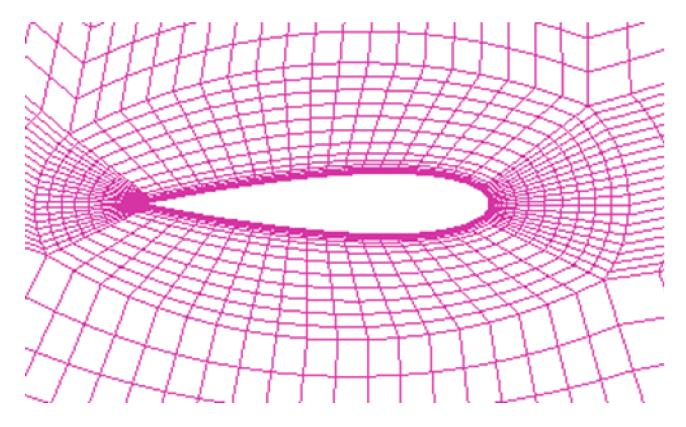Fig.1
Grid distributions around the single blade to control Y + (top view)

The input boundary conditions are: gravity force (g=9.8 m/s), rotational velocity (ω=3.5, 5.25, and 7.875 rad/s), and fixed support at the blade clamp position and FSI at each blade are applied. Fig. 2a shows inputs defined in ANSYS-MA. The interface between ANSYS and CFX is possible due to FSI command. The data exchange occurs at an external boundary in CFX-solver, with mesh displacement defined by the ANSYS multi-field coupling process. After using this command in ANSYS-MA, the interface boundary is created for each blade, i.e., FSIN_1, FSIN_2, FSIN_3, and FSIN_4 for blade 1, 2, 3, and 4, respectively. Later, in CFX-Pre, each blade is considered correspondingly for matching the fluid solid interface. Due to these specifications, CFX-solver transfers forces to ANSYS solver and ANSYS transfers total mesh displacement to the CFX at the interface boundary surface.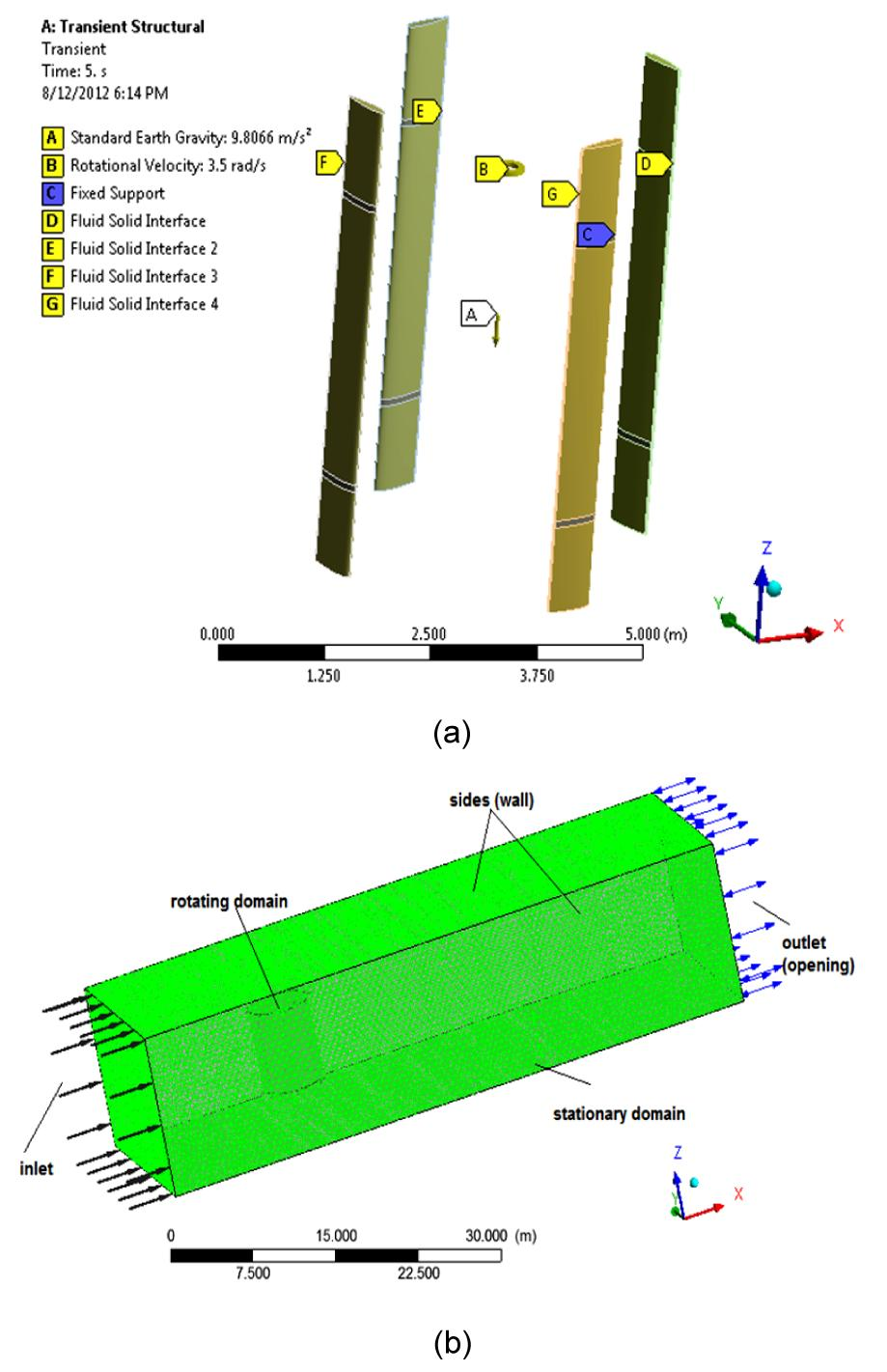Fig.2
Transient structural physics (a) and CFX simulation model with boundary conditions (b)

Next, the analysis setting is reset for a total time of 5 s with time step option of 0.01 s and the program control solver is selected. This is an optimal solver. Because the other solver, i.e., the direct solver, is used for thin flexible models, and the iterative solver is used for bulky models.

### 4.2.  Fluid flow (CFX) physics and modeling

We will attach fluid flow CFX window with transient structural window. All the physics and data will be shared with this window. Here the solid bodies, blades are suppressed and only fluid bodies i.e., rotating, and outer domains are meshed with the following properties: solver preference CFX, use advanced size function (curvature), smoothing (medium), transition (slow), and span angle center (fine). These mesh properties are enough to solve this model. The mesh size will increase with the increase of computational time.

All the boundary conditions (inlet, outlet, sides, rotating, and outer domains) are defined using named selection option in CFX setup. Later in CFX-Pre boundaries, the matching name will be identified which are defined in the setup. Two domains, i.e., stationary and rotating domains in CFX-Pre are created. The rotary domain will be set for a different angular velocity with mesh deformation options of ‘region of motion specified’. The mesh deformation option enables the nodes on the boundary of the mesh using CFX expression language (CEL). The motion of all the remaining nodes will be determined by the mesh motion model, presently the mesh displacement diffusion. This model will be used to transfer the displacement applied at the boundary surface to the other nodes. With this option, a mesh displacement equation will be solved at the start of the iteration. The mesh coordinates will be updated (). Separate boundaries for each blade with boundary type ‘wall’ is defined with mass and momentum option of ‘no-slip wall’. This condition is by default, indicating that the fluid sticks to the wall and moves with the same velocity at the wall; as in this case, blades of turbines rotate. Coupling time duration of 5 s with time step of 0.01 s and transient analyses are also defined in this simulation. Analysis is performed at two times higher than actual velocity, where an inlet boundary condition of normal speed 3.5 m/s is defined in the stationary domain. If the turbine runs safely at this high-current velocity, it will work smoothly at an actual velocity of 1.2 m/s. Furthermore, outlet and symmetry boundary conditions are also described in this domain. Fig. 2b shows CFX simulation model with defined boundary conditions (BC).

When the solution starts running, CFX solver window opens automatically. The solver window exhibits the difference between one-way and two-way coupled simulation. Figs. 3a and 3b show the differences between two simulations, while running in CFX solver manager. It includes ‘ANSYS Field Solver’ (structural) window for forces and ‘ANSYS Interface Load Transfer’ window. These two windows do not appear in case of one-way FSI solver managers. The FSI simulation with the loads described above is run for 500 iterations. These iterations are enough to reach a steady-state condition. Three simulations are run at different angular velocities but with the same BC and with an inlet velocity of 3.5 m/s.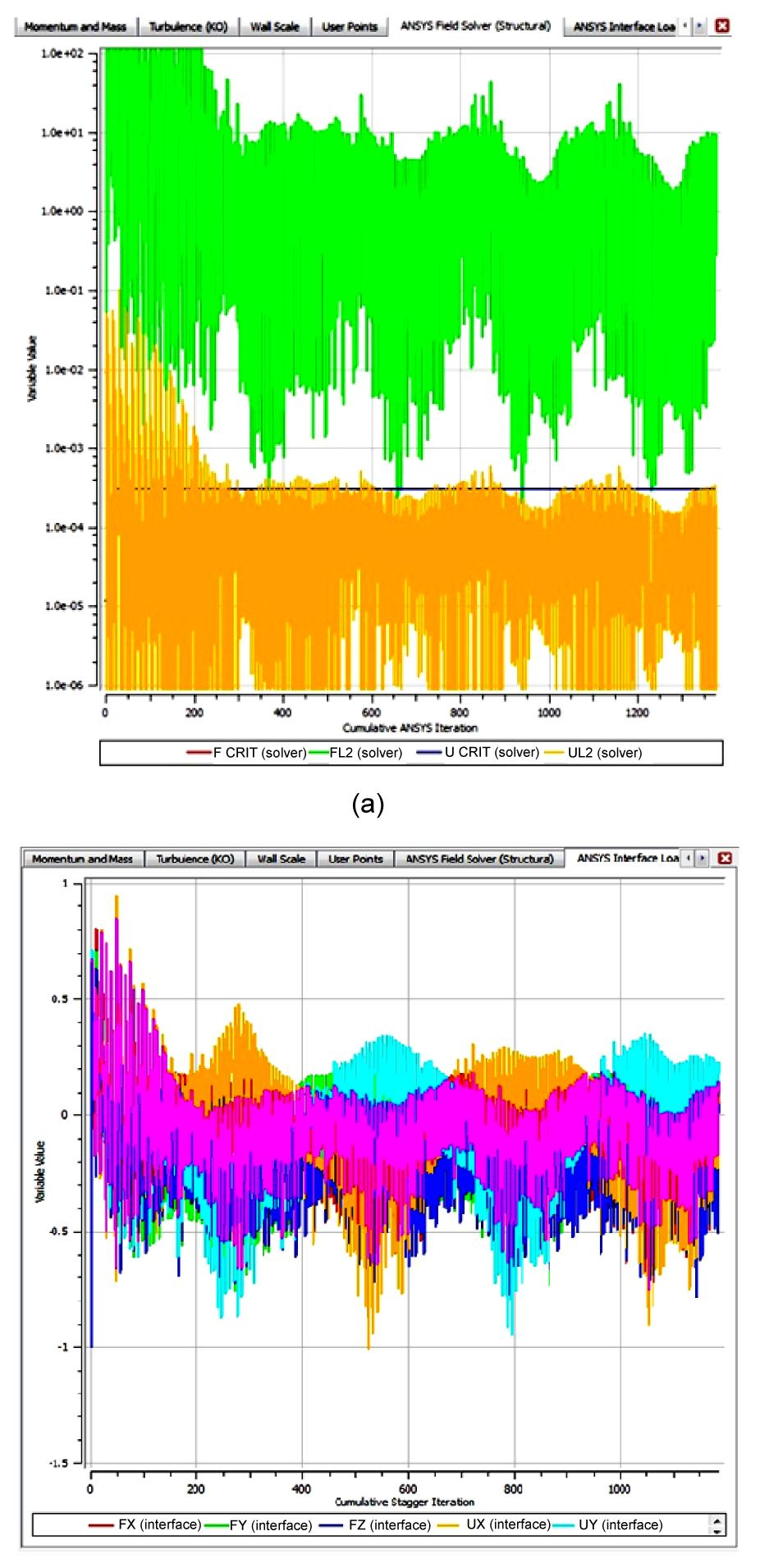Fig.3
Two-way FSI solution running in solver manager (a) and forces and displacement interface window (b)

Figs. 4a and 4b show CFX results for total mesh displacement and velocity vectors. We observed that the entire mesh displacement from CFX (0.004059 m) and total deformation from the ANSYS (0.004616 m) results are similar. This means that numerical simulation was done precisely, and both solvers finished at the same time. Furthermore, this simulation method was validated with ANSYS-13 case examples 22 and 23. Figs. 57 show the ANSYS von-Mises stress and deformations at different angular velocities. From Table 2 (p.581), it can be observed that with an increase in angular velocity, stresses and deformation increase. Greater values of angular velocities were selected, because if blades sustain at higher values, they will perform safely and well at smaller values of ω. Maximum stress calculated was 38 MPa at ω=7.875 rad/s, which are under the limit as the tensile yield strength is 250 MPa, and the ultimate strength is 460 MPa for the steel selected. Fig. 8 exhibits that two-way FSI has higher values of stresses as compared to one-way FSI with an increase in angular velocity.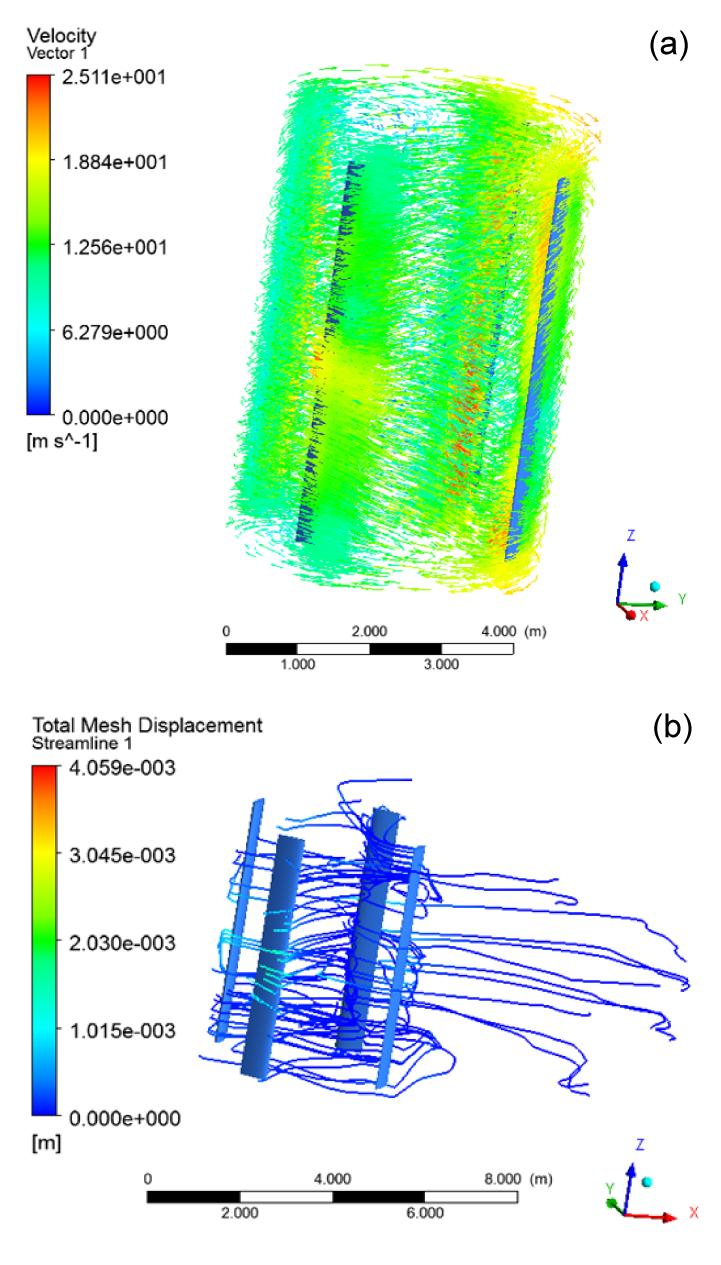Fig.4
CFX results for velocity (m/s) (a) and total mesh displacement (m) (b)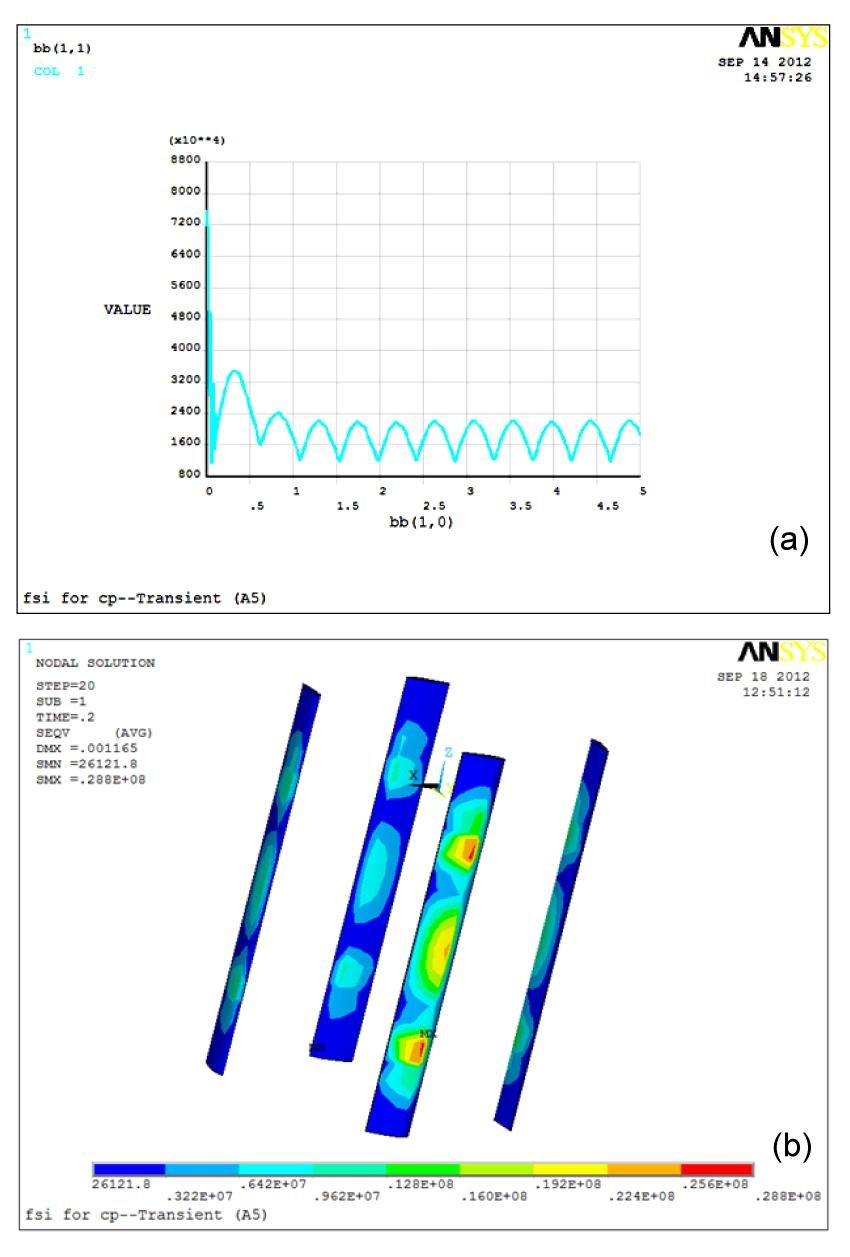Fig.5
ANSYS iteration result (a) and von-Mises stresses and deformation at ω=3.5 rad/s (b)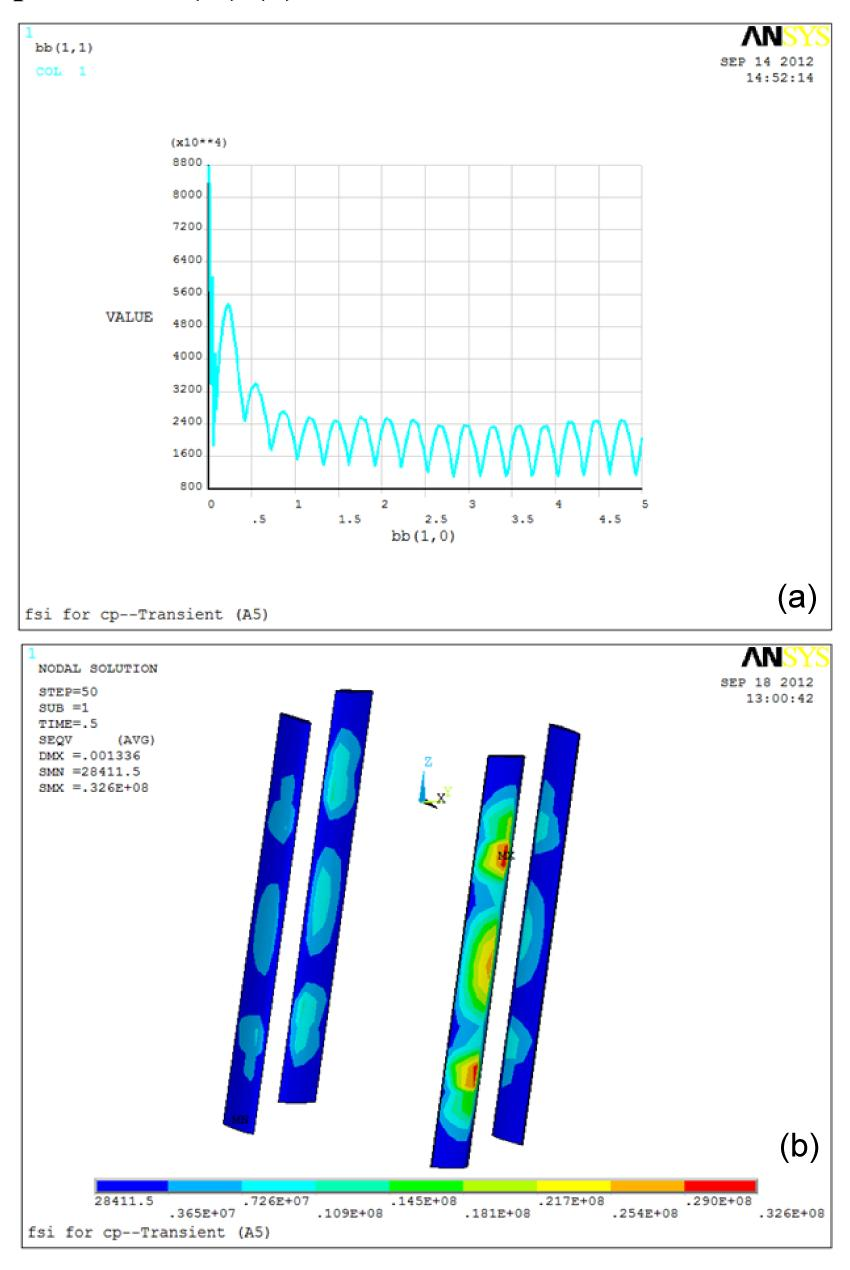Fig.6
ANSYS iteration result (a) and von-Mises stress and deformation at ω=5.25 rad/s (b)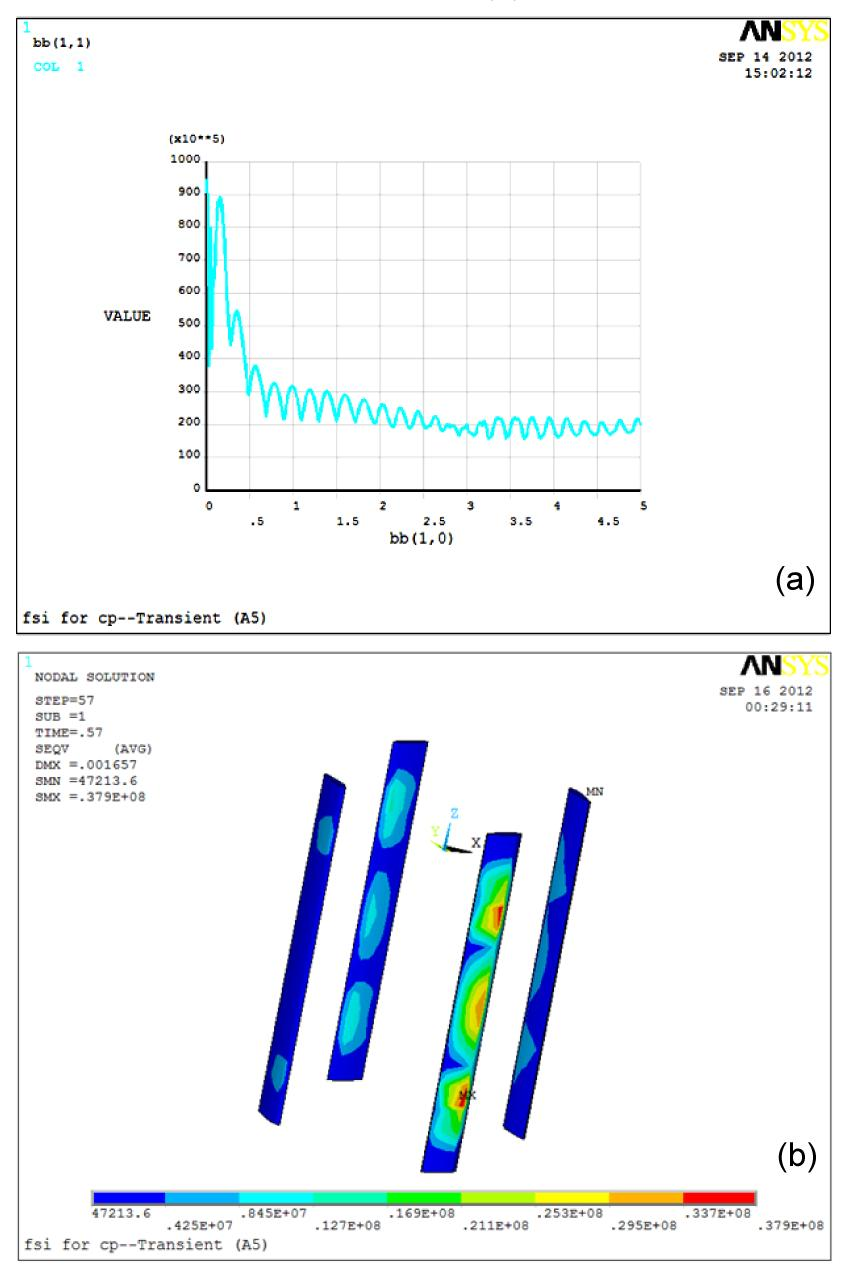Fig.7
ANSYS iteration result (a) and von-Mises stress and deformation at ω=7.875 rad/s (b)

#### Table 2

Difference between two-way and one-way FSI results
 Tip speed ratio, λ=ωR/V Angular velocity, ω (rad/s) Two-way FSI One-way FSI ANSYS stresses, σ (MPa) CFX total mesh displacement (m) ANSYS stresses, σ (MPa) CFX total mesh displacement (m) 2 3.5 28.8 0.001465 24.83 0.002453 3 5.25 32.6 0.003523 26.08 0.003431 4.5 7.875 37.9 0.004059 29.00 0.003542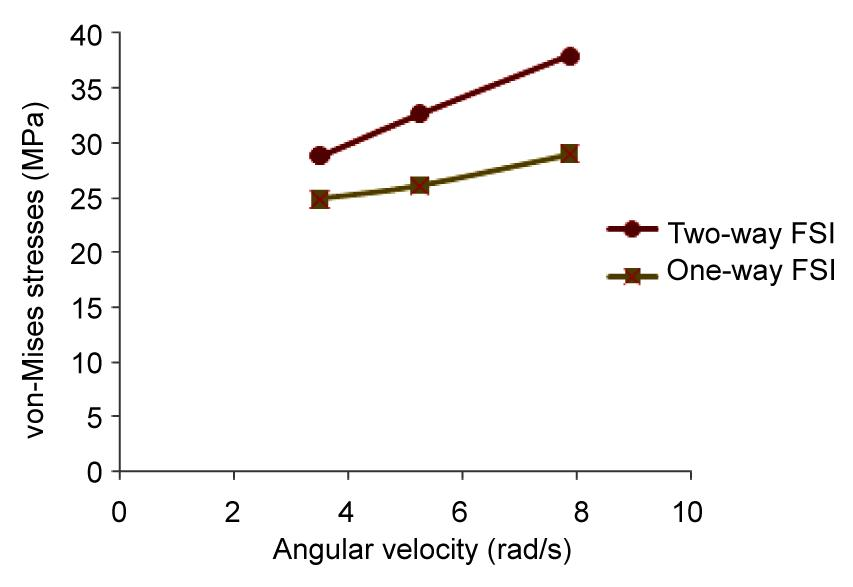Fig.8
Difference between two-way and one-way FSI for stresses versus angular velocity

CFX results for the coefficient of performance against tip speed ratio is calculated and compared with the experimental results obtained by Li () as shown in Fig. 9. For one-way FSI C P=0.432 and for two-way FSI C P=0.4021 at λ=2, and for experimental results C P=0.38 (Li, ). We can observe that the one-way FSI results are far away from the experimental results, and the two-way simulation results are closer with its experimental calculations.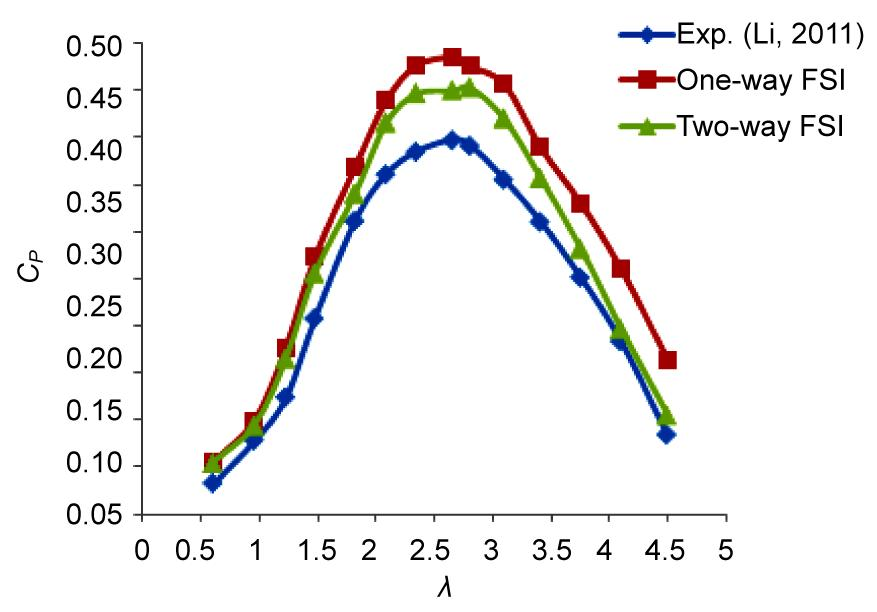Fig.9
Comparison of simulation results with experimental data

## 5.  Conclusions

In this study, a two-way FSI method is used for an actual simulation case study. Due to high experimental cost and complex design of VATT rotor, an accurate and credible method of numerical simulation is preferable. The proposed coupled (two-way) FSI approach is sufficiently precise and certain, as compared to non-coupled (one-way) FSI, especially for large deflection problems where the structure deformation is more visible due to the strong effect of the fluid field. Furthermore, in one-way FSI approach, there is the possibility of lost data between two fields during data transfers. Therefore, we can use one-way or two-way FSI techniques based on the case considered. It depends upon the importance and case sensitivity. If it is an expensive and delicate model, then the two-way FSI is more suitable, otherwise not. Moreover, the one-way FSI requires data for a single iteration per time step. Thus, the computational cost per time step of one-way FSI is lower than that of two-way FSI, which is updated after each time step for a new iteration. People avoid using two-way FSI process, because it takes more computational power and resources. However, the strategy presented in this study can be used to reduce these concerns. In addition, this method makes it possible to use a simple computer for simulation purposes instead of high specification computer.

Therefore, the two-way coupled approach seems to be more stable and accurate, because a large number of time steps can be used for non-coupled method to achieve the same level of accuracy. RANS-based models are more reliable as compared to the vortex method and boundary element method and thus, can be used for design purposes (Turnoak and Wright, ; Li and Calisal, ).

* Project supported by the National Natural Science Foundation of China (Nos. 51209060 and 51106034), the ‘111’ Project Foundation from Ministry of Education and State Administration of Foreign Experts Affairs (No. B07019), China, and the National Special Foundation for Ocean Energy (No. GHME2010CY01)

## ReferencesANSYS CFX Release 13.0 Help, . Convergence Results and RMS (4.2), Mesh Deformation (1.2.2) and Region of Motion Specified (1.2.2.1). Mechanical User Guide, Documentation ANSYS Europe, Ltd,:Benra, F.K., 2006. Numerical and experimental investigation on the flow induced oscillations of a single blade pump impeller. Journal of Fluids Engineering, 128(4):783-793.Benra, F.K., Dohmen, H.J., Pei, J., Schuster, S., Wan, B., 2011. A comparison of one-way & two-way coupling methods for numerical analysis of fluid-structure interactions. Journal of Applied Mathematics, Article ID 853560,:Calcagno, G., Salvatore, F., Greco, L., 2006. Experimental and Numerical Investigation of an Innovative Technology for Marine Current Exploitation: the Kobold Turbine. , Proceedings of the ISOPE Conference, San Francisco, USA, 323:323Casadei, F., Halleux, J.P., Sala, A., Chille, F., 2001. Transient fluid structure interaction algorithms for large industrial applications. Journal of Computer Methods in Applied Mechanics & Engineering, 190(24-25):3081-3110.Dang, H., Yang, Z., Li, Y., 2010. Accelerated loosely-coupled CFD/CSD method for nonlinear static aeroelastic analysis. Journal of Aerospace Science and Technology, 14(4):250-258.Dobrev, I., Massouh, F., 2007. Fluid-structure Interaction in the Case of a Wind Turbine Rotor. , 18me Congress Franais de Mcanique, Grenoble, 27-31. :27-31.Fabio, N., 2010. , International Summer School on Mathematical Modeling & Computation LSEC, Beijing, China, :Garelli, L., Paz, R.R., Storti, M.A., 2010. Fluid structure interaction of the start-up of a rocket engine nozzle. Journal of Computers and Fluids, 39(7):1208-1218.Hubner, B., Walhorn, E., Dinkler, D., 2004. A monolithic approach to fluid structure interaction using space time finite elements. Journal of Computer Methods in Applied Mechanics and Engineering, 193(23-26):2087-2104.Hyman, J.M., Knapp, R., Scovel, J.C., 1992. High order finite volume approximations of differential operators on non-uniform grids. Journal of Physica, D60:112-138.Holger, W., 2008. Hybrid methods for fluid-structure interaction problems in aeroelasticity. Meshfree Methods for Partial Differential Equations IV, 65:335-358.Hbner, B., Seidel, U., Roth, S., 2010. Application of Fluid-structure Coupling to Predict the Dynamic Behavior of Turbine Components. , 25th Symposium on Hydro Machinery & Systems, Romania, :Jo, C.H., Kim, D.Y., Rho, Y.H., Lee, K.H., Johnstone, C., 2012. FSI analysis of deformation along offshore pile structure for tidal current power. Journal of Renewable Energy, 54:248-252.Kim, Y.G., Kim, K.C., 2006. Analysis of fluid structure interaction on 100kW-HAWT-blade. The Korean Society of Visualization, 4(1):41-46.Li, Y., Calisal, S.M., 2010. Three-dimensional effects and arm effects on modeling a vertical axis tidal current turbine. Journal of Renew Energy, 35(10):2325-2334.Li, Z.C., 2011.  Numerical Simulation and Experimental Study on Hydrodynamic Performances of Vertical Axis Tidal Turbine. PhD Thesis, (in Chinese), Harbin Engineering University,China :Menter, F.R., 1994. Two-equation eddy-viscosity turbulence models for engineering applications. AIAA Journal, 32(8):1598-1605.Ouahiba, G., Aziz, H., Anas, S., 2008. Fluid structure interaction of wind turbine airfoils. Journal of Wind Engineering, 32(6):539-557.Paraschivoiu, I., 2002.  Wind Turbine Design. Polytechnic International Press,UK :Ramji, K., Wei, S., 2004. Fluid-structure interaction for aeroelastic applications. Progress in Aerospace Sciences, 40(8):535-558.Turnoak, S.R., Wright, A.M., 2000. Directly coupled fluid structural model of a ship rudder behind a propeller. Journal of Marine Structure, 13(1):53-72.Vaassen, J.M., DeVincenzo, P., Hirsch, C., 2011. Strong Coupling Algorithm to Solve Fluid Structure-interaction Problems with a Staggered Approach. , Proceedings of the 7th European Symposium on Aerothermodynamics, the Netherlands, :Wei, S.H., Zhang, L.J., Yan, Y.Z., 2009. Research on Aero-elasticity of Horizontal Axis Wind Turbine by a Fluid-structure Coupling Numerical Method. , Proceedings of the IEEE International Conference on Sustainable Power Generation & Supply, Nanjing, China, 1-5. :1-5.Zanette, J., Imbault, D., Tourabi, A., 2010. A design methodology for cross flow water turbines. Journal of Renew Energy, 35(5):997-1009.Zhang, L., Wang, L.B., Li, F.L., 2004. Study on stream tube models for prediction performance of vertical-axis variable-pitch turbine for tidal current energy conversion. Journal of Harbin Engineering University, 25(3):261-266.